Math Notes For Class 12 Download PDF Continuity And Differentiability Chapter 5

Chapter 5: Continuity and Differentiability

## Chapter 5: Continuity and Differentiability

### Derivative

The rate of change of a quantity y with respect to another quantity x is called the derivative or differential coefficient of y with respect to x .

### Differentiation of a Function

Let f(x) is a function differentiable in an interval [a, b]. That is, at every point of the interval, the derivative of the function exists finitely and is unique. Hence, we may define a new function g: [a, b] → R, such that, ∀ x ∈ [a, b], g(x) = f'(x).
This new function is said to be differentiation (differential coefficient) of the function f(x) with respect to x and it is denoted by df(x) / d(x) or Df(x) or f'(x).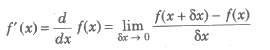### Differentiation ‘from First Principle

Let f(x) is a function finitely differentiable at every point on the real number line. Then, its derivative is given by#### Standard Differentiations

1. d / d(x) (xn) = nxn – 1, x ∈ R, n ∈ R
2. d / d(x) (k) = 0, where k is constant.
3. d / d(x) (ex) = ex
4. d / d(x) (ax) = ax loge a > 0, a ≠ 1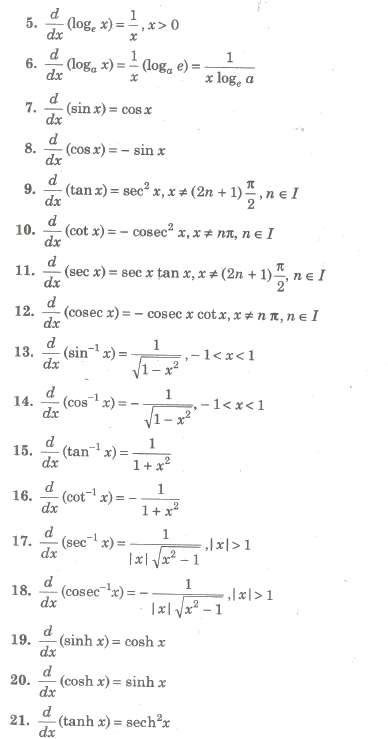#### Fundamental Rules for Differentiation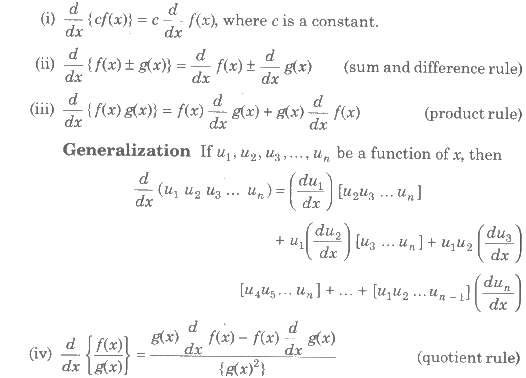(v) if d / d(x) f(x) = φ(x), then d / d(x) f(ax + b) = a φ(ax + b)
(vi) Differentiation of a constant function is zero i.e., d / d(x) (c) = 0.

#### Geometrically Meaning of Derivative at a Point

Geometrically derivative of a function at a point x = c is the slope of the tangent to the curve y = f(x) at the point {c, f(c)}.
Slope of tangent at P = lim x → c f(x) – f(c) / x – c = {df(x) / d(x)} x = c or f’ (c).

##### Different Types of Differentiable Function
###### 1. Differentiation of Composite Function (Chain Rule)

If f and g are differentiable functions in their domain, then fog is also differentiable and
(fog)’ (x) = f’ {g(x)} g’ (x)
More easily, if y = f(u) and u = g(x), then dy / dx = dy / du * du / dx.
If y is a function of u, u is a function of v and v is a function of x. Then, dy / dx = dy / du * du / dv * dv / dx.

###### 2. Differentiation Using Substitution

In order to find differential coefficients of complicated expression involving inverse trigonometric functions some substitutions are very helpful, which are listed below .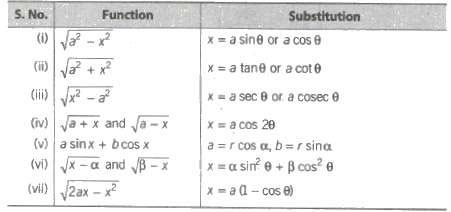###### 3. Differentiation of Implicit Functions

If f(x, y) = 0, differentiate with respect to x and collect the terms containing dy / dx at one side and find dy / dx.
Shortcut for Implicit Functions For Implicit function, put d /dx {f(x, y)} = – ∂f / ∂x / ∂f / ∂y, where ∂f / ∂x is a partial differential of given function with respect to x and ∂f / ∂y means Partial differential of given function with respect to y.

###### 4. Differentiation of Parametric Functions

If x = f(t), y = g(t), where t is parameter, then dy / dx = (dy / dt) / (dx / dt) = d / dt g(t) / d / dt f(t) = g’ (t) / f’ (t)

###### 5. Differential Coefficient Using Inverse Trigonometrical Substitutions

Sometimes the given function can be deducted with the help of inverse Trigonometrical substitution and then to find the differential coefficient is very easy.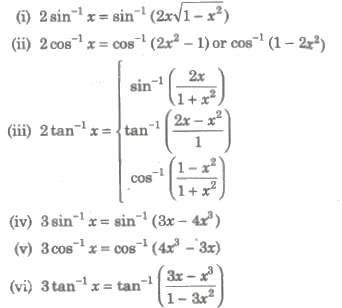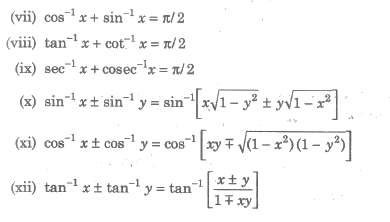#### Logarithmic Differentiation Function

(i) If a function is the product and quotient of functions such as y = f1(x) f2(x) f3(x)… / g1(x) g2(x) g3(x)… , we first take algorithm and then differentiate.
(ii) If a function is in the form of exponent of a function over another function such as [f(x)]g(x) , we first take logarithm and then differentiate.

#### Differentiation of a Function with Respect to Another Function

Let y = f(x) and z = g(x), then the differentiation of y with respect to z is dy / dz = dy / dx / dz / dx = f’ (x) / g’ (x)

#### Successive Differentiations

If the function y = f(x) be differentiated with respect to x, then the result dy / dx or f’ (x), so obtained is a function of x (may be a constant).
Hence, dy / dx can again be differentiated with respect of x.
The differential coefficient of dy / dx with respect to x is written as d /dx (dy / dx) = d2y / dx2 or f’ (x). Again, the differential coefficient of d2y / dx2 with respect to x is written as d / dx (d2y / dx2) = d3y / dx3 or f”'(x)……
Here, dy / dx, d2y / dx2, d3y / dx3,… are respectively known as first, second, third, … order differential coefficients of y with respect to x. These alternatively denoted by f’ (x), f” (x), f”’
(x), … or y1, y2, y3…., respectively.
Note dy / dx = (dy / dθ) / (dx / dθ) but d2y / dx2 ≠ (d2y / dθ2) / (d2x / dθ2)

#### Leibnitz Theorem

If u and v are functions of x such that their nth derivative exist, then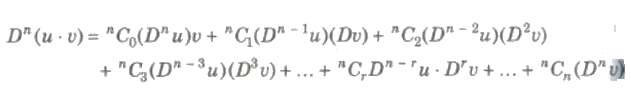#### nth Derivative of Some Functions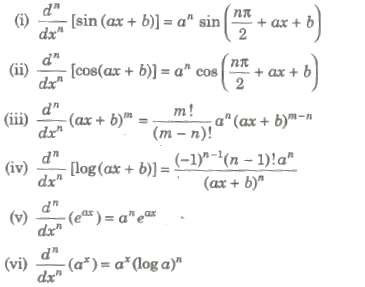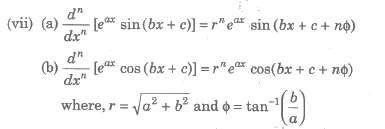#### Derivatives of Special Types of Functions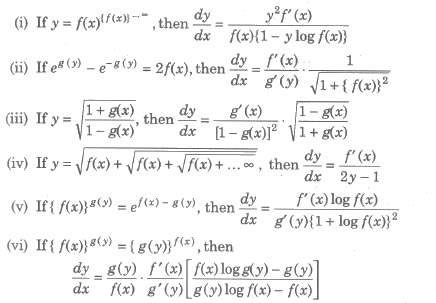##### (vii) Differentiation of a Determinant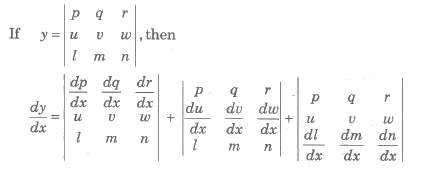##### (viii) Differentiation of Integrable Functions

If g1 (x) and g2 (x) are defined in [a, b], Differentiable at x ∈ [a, b] and f(t) is continuous for g1(a) ≤ f(t) ≤ g2(b), then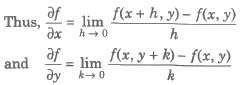##### Partial Differentiation

The partial differential coefficient of f(x, y) with respect to x is the ordinary differential coefficient of f(x, y) when y is regarded as a constant. It is a written as ∂f / ∂x or Dxf or fx.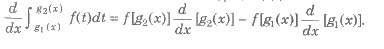e.g., If z = f(x, y) = x4 + y4 + 3xy2 + x4y + x + 2y
Then, ∂z / ∂x or ∂f / ∂x or fx = 4x3 + 3y2 + 2xy + 1 (here, y is consider as constant) ∂z / ∂y or ∂f / ∂y or fy = 4y3 + 6xy + x2 + 2 (here, x is consider as constant)

##### Higher Partial Derivatives

Let f(x, y) be a function of two variables such that ∂f / ∂x , ∂f / ∂y both exist.

(i) The partial derivative of ∂f / ∂y w.r.t. ‘x’ is denoted by ∂2f / ∂x2 / or fxx.
(ii) The partial derivative of ∂f / ∂y w.r.t. ‘y’ is denoted by ∂2f / ∂y2 / or fyy.
(iii) The partial derivative of ∂f / ∂x w.r.t. ‘y’ is denoted by ∂2f / ∂y ∂x / or fxy.
(iv) The partial derivative of ∂f / ∂x w.r.t. ‘x’ is denoted by ∂2f / ∂y ∂x / or fyx.

Note ∂2f / ∂x ∂y = ∂2f / ∂y ∂x
These four are second order partial derivatives.

##### Euler’s Theorem on Homogeneous Function

If f(x, y) be a homogeneous function in x, y of degree n, then x (&partf / ∂x) + y (&partf / ∂y) = nf

##### Deduction Form of Euler’s Theorem

If f(x, y) is a homogeneous function in x, y of degree n, then
(i) x (∂2f / ∂x2) + y (∂2f / ∂x ∂y) = (n – 1) &partf / ∂x
(ii) x (∂2f / ∂y ∂x) + y (∂2f / ∂y2) = (n – 1) &partf / ∂y
(iii) x2 (∂2f / ∂x2) + 2xy (∂2f / ∂x ∂y) + y2 (∂2f / ∂y2) = n(n – 1) f(x, y)

##### Important Points to be Remembered

If α is m times repeated root of the equation f(x) = 0, then f(x) can be written as f(x) =(x – α)m g(x), where g(α) ≠ 0.
From the above equation, we can see that f(α) = 0, f’ (α) = 0, f” (α) = 0, … , f(m – l) ,(α) = 0.
Hence, we have the following proposition f(α) = 0, f’ (α) = 0, f” (α) = 0, … , f(m – l) ,(α) = 0.
Therefore, α is m times repeated root of the equation f(x) = 0.

### NCERT Books Free Pdf Download for Class 5, 6, 7, 8, 9, 10 , 11, 12 Hindi and English Medium

 Mathematics Biology Psychology Chemistry English Economics Sociology Hindi Business Studies Geography Science Political Science Statistics Physics Accountancy